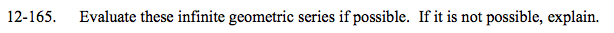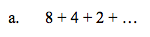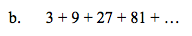### Home > A2C > Chapter 12 > Lesson 12.4.1 > Problem12-165

12-165.Find the common ratio.

$\frac{4}{8}=\frac{1}{2}$

$\frac{2}{4}=\frac{1}{2}$

Use the equation for an infinite geometric series:

$\sum_{n=1}^{\infty}ar^{n-1}=\frac{a}{1-r}$

16See part (a).

Not possible. r > 1, and the terms keep increasing.